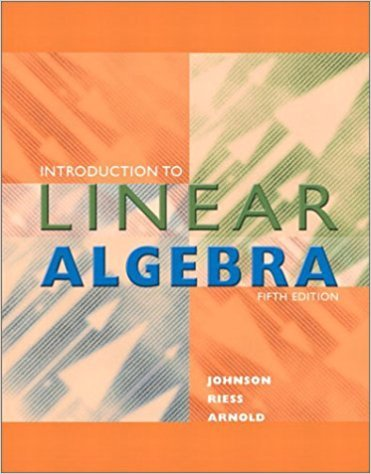×
×

# Consider the two functionsy = cos 2x + sin xandy = 0.01ISBN: 9780201658590 59

## Solution for problem 2E Chapter A.4

Introduction to Linear Algebra | 5th Edition

• Textbook Solutions
• 2901 Step-by-step solutions solved by professors and subject experts
• Get 24/7 help from StudySoup virtual teaching assistantsIntroduction to Linear Algebra | 5th Edition

4 5 1 265 Reviews
25
2
Problem 2E

Consider the two functions

y = cos 2x + sin x

and

y = 0.01 tanx

where

0 < = x < = 9

We want to estimate the points where the graphs of these two functions intersect. To that end, prepare an xy-table of values, where x = 0.0,0.1, 0 .2 ,..., 9.0 and y = cos 2x + sin x.

a) Graph the curve you have just defined.

b) Superimpose the curve defined by y=0.01 tan x, coloring this latter curve red.

c) Note that there appear to be six places where these two curves intersect. Argue that, in reality, there can be only four points where the graphs of the two functions intersect. Can you give a reason for the two “extraneous” intersection points?

d) Estimate the (x , y) coordinates of the actual intersection points.

Step-by-Step Solution:
Step 1 of 3

Intro to Sociology Notes Symbolic Interactionism  Creation and interpretation of symbols  Humans unable to interact in meaningful ways without using symbols  Humans attach meaning to things, places, situations, movements, positions, and more  Symbols allow us to interpret-more or less accurately- the thoughts, feelings, and intentions of those with...

Step 2 of 3

Step 3 of 3

##### ISBN: 9780201658590

Unlock Textbook Solution